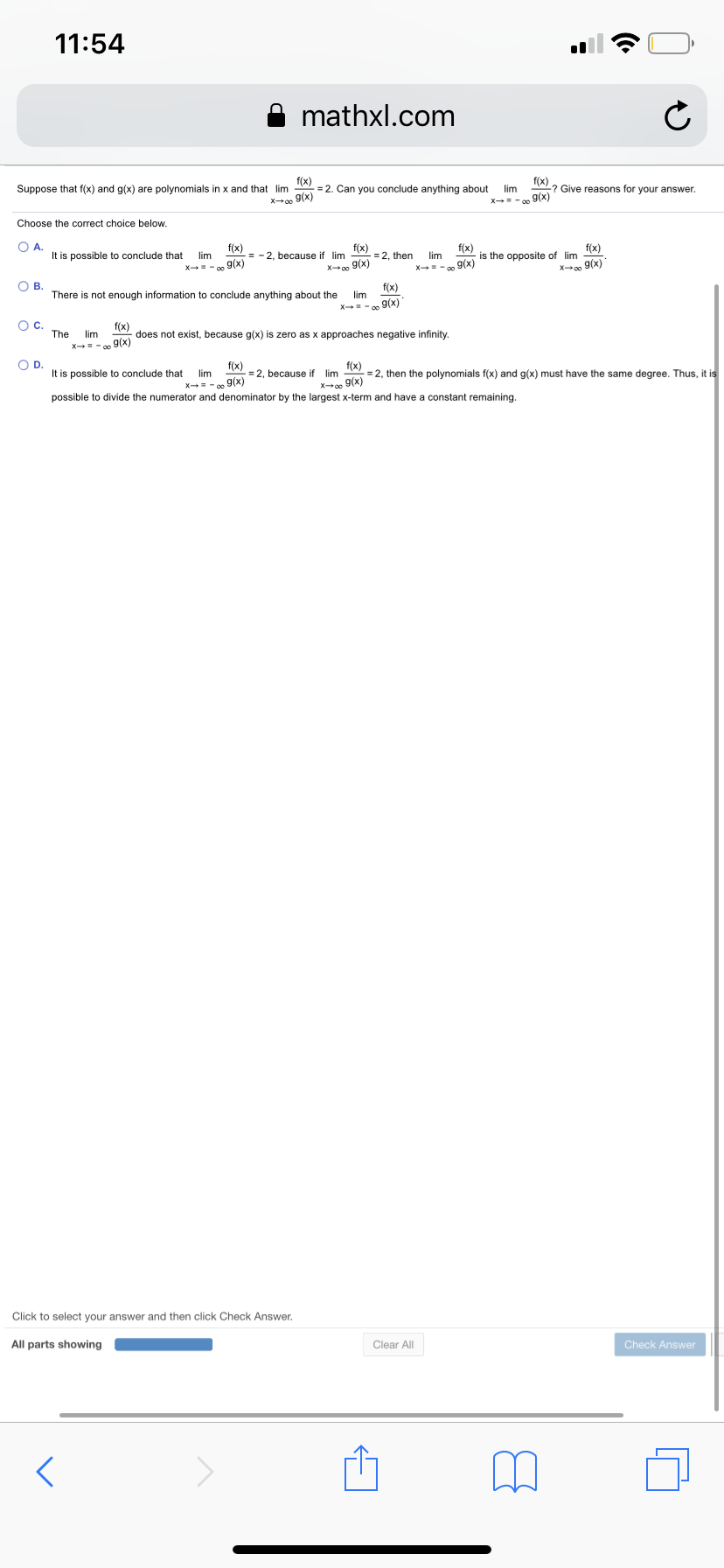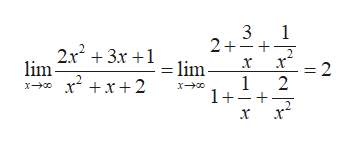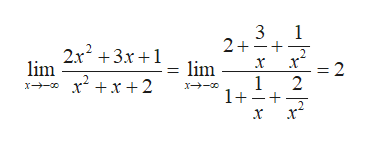11:54mathxl.comSuppose that f(x) and g(x) are polynomials in x and that im r)2. Can you conclude anything aboutx? Give reasons for your answer.9(x)xo0Choose the correct choice below.O A.It is possible to conclude thatf(x= -2, because if limf(x)fixis the opposite of limx g(X)=2, thenx 9(x)O B.There is not enough information to conclude anything about thef(x)lim9(x)O CThef(x)imx 9/y) does not exist, because g(x) is zero as x approaches negative infinity.O D.It is possible to conclude thatf(x)=2, because iff(x)2, then the polynomials f(x) and g(x) must have the same degree. Thus, it isx 9x)possible to divide the numerator and denominator by the largest x-term and have a constant remaining.Click to select your answer and then click Check Answer.All parts showingClear AllCheck Answer

Question

Can you help me step by step with this problem?help_outlineImage Transcriptionclose11:54 mathxl.com Suppose that f(x) and g(x) are polynomials in x and that im r)2. Can you conclude anything about x ? Give reasons for your answer. 9(x) xo0 Choose the correct choice below. O A. It is possible to conclude that f(x = -2, because if lim f(x) fix is the opposite of lim x g(X) =2, then x 9(x) O B. There is not enough information to conclude anything about the f(x) lim 9(x) O C The f(x) im x 9/y) does not exist, because g(x) is zero as x approaches negative infinity. O D. It is possible to conclude that f(x) =2, because if f(x) 2, then the polynomials f(x) and g(x) must have the same degree. Thus, it is x 9x) possible to divide the numerator and denominator by the largest x-term and have a constant remaining. Click to select your answer and then click Check Answer. All parts showing Clear All Check Answer fullscreen
Step 1

Here, f(x) and g(x) are polynomials in x such that:

Step 2

Since, the limit of the fraction is a constant, so both the polynomial must have same degree because then it will be possible to divide the numerator and denominator by the largest x-term and have a constant remaining.

For example: take f(x) = 2x2+3x+1 and g(x) = x2+x+2

Now, here,help_outlineImage Transcriptionclose3 1 2++ +3x+1lim x =2 lim x2x2 1 2 1++ fullscreen
Step 3

Now, similarly limit at negative infi...help_outlineImage Transcriptionclose3 2+ 1 232+3x+1 lim lim x2x2 x - =2 1 2 1++ x + fullscreen

Want to see the full answer?

See Solution

Want to see this answer and more?

Our solutions are written by experts, many with advanced degrees, and available 24/7

See Solution
Tagged in

Limits